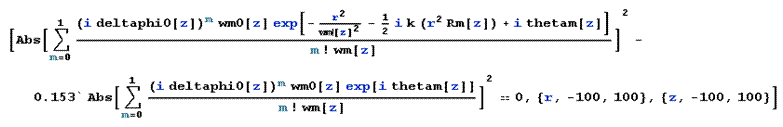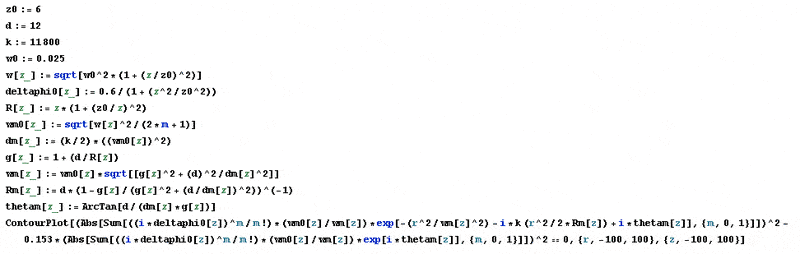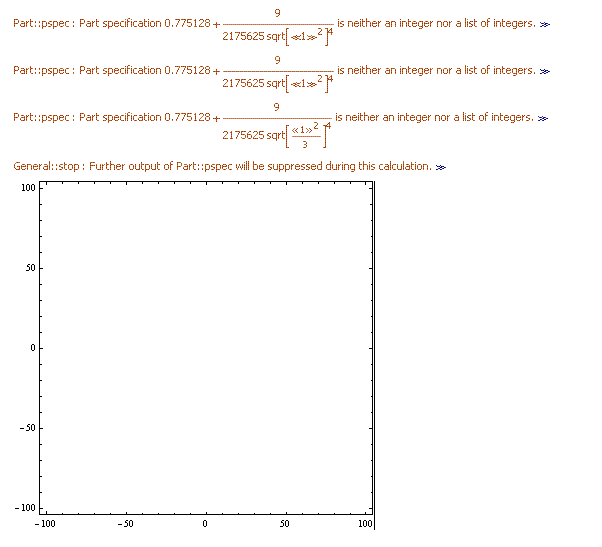# Mathematica, Plot Implicit function contain Sum (sigma)

• Mathematica
Dear friends,
Over the past week, I tried to plot implicit function by mathematica but failed. I am very disappointed. Hopefully someone help me at this time.
My equation is given by (see below figure):Where
z0 := 6
d := 12
k := 11800
w0 := 0.025
w[z_] := sqrt[w0^2*(1 + (z/z0)^2)]
deltaphi0[z_] := 0.6/(1 + (z^2/z0^2))
R[z_] := z*(1 + (z0/z)^2)
wm0[z_] := sqrt[w^2/(2*m + 1)]
dm[z_] := (k/2)*((wm0)^2)
g[z_] := 1 + (d/R)
wm[z_] := wm0*sqrt[[g^2 + (d)^2/dm^2]]
Rm[z_] := d*(1 - g/(g^2 + (d/dm)^2))^(-1)
thetam[z_] := ArcTan[d/(dm*g)]
I want to plot a graph to represent the dependence of r (z). I have tried the following code-mathematica fileBut mathamatica plot 2 coordinate axes without data (no points).Please help me!
This is the mathematica file
https://docs.google.com/a/hcmus.edu.vn/file/d/0B6ue0CPI1AOFcWpjd19zMEU0a2s/edit
and my function
https://docs.google.com/a/hcmus.edu.vn/file/d/0B6ue0CPI1AOFTXMxRFZDaXd6NjQ/edit
Thanks very much.

## Answers and Replies

Quick look suggest you got that little s in there: First rule of Mathematica: all built-in functions names start with capital letters like Sqrt

I don't know how many more errors remain, but this fixes the first couple of waves and does plot something.

In:= z0 = 6;
d = 12;
k = 11800;
w0 = 0.025;
w[z_] := Sqrt[w0^2*(1 + (z/z0)^2)];
deltaphi0[z_] := 0.6/(1 + (z/z0)^2);
R[z_] := z*(1 + (z0/z)^2);
wm0[z_] := Sqrt[w[z]^2/(2*m + 1)];
dm[z_] := k/2*wm0[z]^2;
g[z_] := 1 + d/R[z];
wm[z_] := wm0[z]*Sqrt[g[z]^2 + (d/dm[z])^2];
Rm[z_] := d/(1 - g[z]/(g[z]^2 + (d/dm[z])^2));
thetam[z_] := ArcTan[d/(dm[z]*g[z])];
ContourPlot[ Abs[ Sum[ (I*deltaphi0[z])^m/m!*wm0[z]/wm[z]* Exp[-r^2/wm[z]^2 - I*k*r^2/2*Rm[z] + I*thetam[z]], {m, 0, 1}]]^2 - 0.153*Abs[ Sum[ (I*deltaphi0[z])^m/m!*wm0[z]/wm[z]*Exp[I*thetam[z]], {m, 0, 1}]]^2 == 0, {r, -100, 100}, {z, -100, 100}]

Out= ...PlotSnipped...

There are fairly clearly other things wrong. For example, when I change your plot range {r, -100, 100} to {r, -5, 5} to try to zoom in on the horizontal axis, instead of getting a substantially wider and more detailed version of your plot I get a narrower and narrower plot. So I think at least a couple of other things are seriously broken in the code.

Last edited:
So I think at least a couple of other things are seriously broken in the code.

Great. That's a good start.

Now, to the poster:

How do we proceed from here? Well, I can tell you how to fix it without even looking at it: Start cutting things out, make it simpler while trying to keep the qualitative content of the code you're trying to run. Keep doing that even if you have to mercilessly hack it to death until it only vaguely resembles the original code. Get that simplified version running. Then piece by piece and not 2 or three at time, add one thing to the code, get it running, add another, get it running, then another until you build it back up to what you're trying to do.